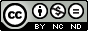Solvmanifolds with holomorphically trivial canonical budle

Otal Germán, Antonio
Ugarte Vilumbrales, Luis (dir.)

Abstract: As it is well-known Calabi-Yau manifolds constitute one of the most important classes in geometry. These manifolds, which can be thought as higher-dimensional analogues of K3 surfaces, are compact complex manifolds (M,J) of complex dimension n endowed with an SU(n) structure (F,\Psi) such that the fundamental 2-form F is closed and the (n,0)-form \Psi is holomorphic. Thus, the holonomy of the metric g reduces to a subgroup of SU(n), so that g is a Ricci-flat Kähler metric, and the canonical bundle of (M,J) is holomorphically trivial. The above conditions defining a Calabi-Yau manifold have been weakened in different directions so that the resulting geometries still play an important role in several aspects of complex geometry. In this thesis we focus our attention in the geometry of compact complex manifolds (M,J) with holomorphically trivial canonical bundle endowed with special Hermitian metrics which are less restrictive than the Kähler ones. Concerning compact complex manifolds with holomorphically trivial canonical bundle, we recall that in complex dimension 2 the possibilities, up to isomorphism, are a K3 surface, a torus or a Kodaira surface, where the first two are Kähler and the latter is an example of a nilmanifold M = G/ \Gamma, i.e. a compact quotient of a simply connected nilpotent Lie group G by a lattice \Gamma of maximal rank in G. However, there are no classifications in complex dimension 3 or higher, so it is natural to begin by studying such complex geometry on some particular classes of compact manifolds of real dimension 6. A good candidate is the class consisting of nilmanifolds endowed with an invariant complex structure, as Salamon proved that any such complex nilmanifold has holomorphically trivial canonical bundle. In (real) dimension 6 a classification of nilmanifolds admitting this kind of complex structures is also provided by Salamon, where the Iwasawa (nil)manifold is a classical example which plays a relevant role in complex geometry. Although the complex geometry of nilmanifolds provides an important source of examples in differential geometry, these spaces never satisfy the ddbar-lemma because they are not formal except for tori. However, the investigation of some properties in complex geometry requires compact complex manifolds satisfying the ddbar-lemma, so one needs to consider a broader class of homogeneous spaces M = G/ \Gamma. The first natural generalization of nilmanifolds is given by compact quotients of Lie groups G which are solvable instead of nilpotent. For instance, the Nakamura manifold, whose complex geometry is very rich, is an example of this type. This class of manifolds, known as solvmanifolds, is the central object of study in this thesis. More concretely, we describe the 6-dimensional solvmanifolds admitting an invariant complex structure with holomorphically trivial canonical bundle, as well as we obtain a classification of such invariant structures. As we mentioned above, another goal in this thesis is the study of special Hermitian metrics which are less restrictive than the Kähler ones. It is well-known that the existence of a Kähler metric on a compact manifold imposes strong topological obstructions. In contrast, Gauduchon proves that on a compact complex manifold (M,J) of complex dimension n there always exists a Gauduchon metric, defined by ddbar F^{n-1}=0, in the conformal class of any given Hermitian metric. Between the Kähler class and the Gauduchon class other interesting classes of special Hermitian metrics have been considered in relation to different problems in differential geometry such as balanced, strongly Gauduchon, strong Kähler with torsion and k-th Gauduchon. Associated to any compact complex manifold (M,J) there exist several complex invariants which measure some specific aspects of (M,J). Among them, we distinguish the Dolbeault, the Bott-Chern and the Aeppli cohomologies, and the Frölicher spectral sequence {E_r(M)}, with r>=1, relating the Dolbeault to the de Rham cohomology of the manifold. If M is a compact Kähler manifold then all these complex invariants coincide because M satisfies the ddbar-lemma, however the Frölicher sequence may not degenerate at the first step for arbitrary compact complex manifolds. A problem of interest in complex geometry is to study the behaviour of these invariants. In the case of 6-dimensional nilmanifolds a complete picture of the behaviour of the sequence {E_r(M)} is given in this thesis, and for solvmanifolds of dimension 6 endowed with an invariant complex structure of splitting type (in the sense of Kasuya) with holomorphically trivial canonical bundle we use the results by Kasuya and Angella and by Angella and Tomassini to find when the ddbar-lemma is satisfied. Finally, motivated by some recent results obtained by Popovici, we also explore in this thesis the relations among the degeneration of the Frölicher spectral sequence, the ddbar-lemma and the existence of balanced or strongly Gauduchon metrics, as well as their behaviour under small holomorphic deformations of the complex structure.

Pal. clave: álgebra de lie ; variedades complejas ; geometría diferencial

Knowledge area: Álgebra

Department: Matemáticas

Nota: Tesis-Univ. Zaragoza, Matemáticas, 2014